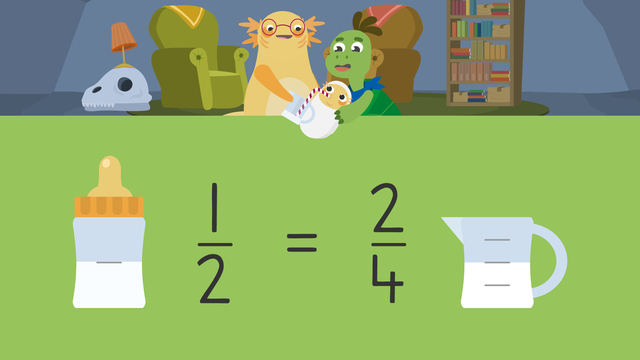# Equivalent FractionsRate this video

Ø 5.0 / 1 ratings

The authorTeam Digital
Equivalent Fractions
CCSS.MATH.CONTENT.3.NF.A.3.B

## Information about the videoEquivalent Fractions

### In This 3rd Grade Equivalent Fractions Video

Axel and Tank are babysitting Axel’s little brother, Odell. Mom left very strict instructions for them to follow. But, she accidentally took the diaper bag and the only bottles. The only containers left in the house have different measurements on them! In this equivalent fractions video for kids, Axel and Tank learn about the meaning of equivalent fractions and how to determine if fractions are equivalent in order to feed Baby Odell correctly.

### What are Equivalent Fractions? An Equivalent Fractions Introduction

Below you will find an in depth explanation of equivalent fractions, examples for teaching equivalent fractions, and examples of equivalent fractions.

#### Equivalent Fractions Definition

A simple definition for equivalent fractions is as follows:

Equivalent fractions are fractions that are from the same whole with numerators and denominators different from one another but identical in value.#### How Can You Tell if Two Fractions are Equivalent?

Models help with how to tell if fractions are equivalent. We can compare the value of different fractions from the same whole using models called fraction bars. Fraction bars show us whether or not the fractions are equivalent, or equal in value. We can draw and label two models that show equivalent fractions.#### What Does it Mean When Two Fractions are Equivalent?

Assuming the fractions are from the same whole, when two fractions are equivalent it means that their numerator and denominator are different from each other but the value of the fraction is the same.

### How to Work Out Equivalent Fractions?

Let’s begin by comparing equivalent fractions such as one-half and two-fourths. To determine if they are equal in value, the first step is to draw equivalent fractions models for both fractions. Represent the first fraction by shading that bar to represent one-half.Then, represent the second fraction on a bar of identical size by shading it to represent two-fourths.Finally, compare the shaded values of both bars to see if fractions are equivalent. Each fraction bar has the same quantity shaded, which means they are equal in value. Since they are equal in value, the fractions one-half and two fourths are equivalent.Let’s try one more example using four-sixths and two-fifths to tell whether the fractions are equivalent. We will see if they are equivalent fractions with models. To determine if they are equal in value, we will draw the fraction bar to represent the first fraction. Shade that fraction bar to represent four-sixths.Then, draw a second fraction bar of identical size to represent the second fraction. Shade that fraction bar to represent two-fifths.Finally, compare the shaded value of both bars. Since the shaded values are different, one half and two fifths are not equivalent fractions.### Summary of Steps: Identifying Equivalent Fractions For Kids

As an introduction to equivalent fractions, we model equivalent fractions in 3rd grade. We can compare the value of different fractions from the same whole using models called fraction bars. Fraction bars show us whether or not the fractions are equivalent, or equal in value. To determine if two fractions from the same whole are equivalent:

• First, draw and shade a fraction bar to represent the first fraction.

• Then, draw and shade another fraction bar of identical size to represent the second fraction.

• Finally, compare the shaded values of both bars.

• If they are equal in value, the fractions are equivalent.

### Now that You’ve Watched the Video on Equivalent Fractions 3rd Grade

Have you done equivalent fractions practice yet? On this website, you can also find 3rd grade equivalent fractions worksheets and exercises.